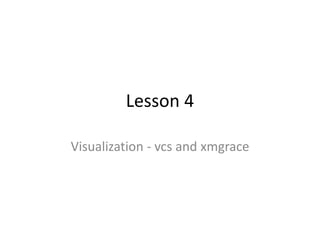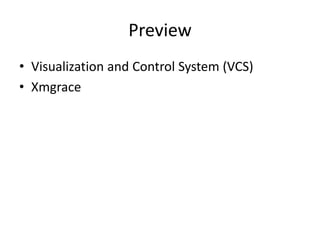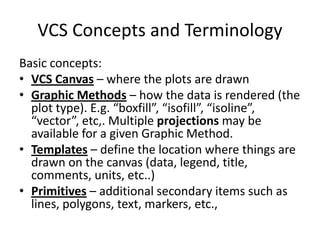Seu SlideShare está sendo baixado. ×

# CDAT - graphics - vcs - xmgrace - Introduction

Anúncio
Anúncio
Anúncio
Anúncio
Anúncio
Anúncio
Anúncio
Anúncio
Anúncio
Anúncio
AnúncioCarregando em…3
×

1 de 31 Anúncio

# CDAT - graphics - vcs - xmgrace - Introduction

This is created by Dr.Krishna Achuta Rao, Centre for Atmospheric Sciences, IIT-Delhi, for taking CDAT class. It's under Creative Common License 3.0

This is created by Dr.Krishna Achuta Rao, Centre for Atmospheric Sciences, IIT-Delhi, for taking CDAT class. It's under Creative Common License 3.0

Anúncio
Anúncio

### CDAT - graphics - vcs - xmgrace - Introduction

1. 1. Lesson 4 Visualization ‐ vcs and xmgrace
2. 2. Preview • Visualization and Control System (VCS) • Xmgrace
3. 3. VCS Concepts and Terminology Basic concepts: • VCS Canvas – where the plots are drawn • Graphic Methods – how the data is rendered (the  plot type). E.g. “boxfill”, “isofill”, “isoline”,  “vector”, etc,. Multiple projections may be  available for a given Graphic Method. • Templates – define the location where things are  drawn on the canvas (data, legend, title,  comments, units, etc..) • Primitives – additional secondary items such as  lines, polygons, text, markers, etc.,
4. 4. The VCS Canvas • VCS canvas needs to be initialized (created)  >>> x=vcs.init() # without any arguments • Up to 8 Canvases at once. • Canvas as “magic board”, can clean or destroy it: >>> x.clear() >>> x.close() • Can have multiple plots on a single Canvas (not  covered here)
5. 5. VCS Help • Basic help on VCS can be obtain inline via: >>> vcs.help() • This will list available function in VCS, notably  functions to create/get/query VCS objects…
6. 6. VCS First Help This also can be used to get help on a specific  command: >>> vcs.help(‘createboxfill’) Function: createboxfill # Construct a new boxfill graphics method Description of Function: Create a new boxfill graphics method given the name and the existing boxfill graphics method to copy the  attributes from. If no existing boxfill graphics  method name is given, then the default boxfill graphics method will be used  as the graphics method to which the attributes will be copied from. If the name provided already exists, then a error will be returned. Graphics  method names must be unique.
7. 7. Graphic Methods Concepts (1) • Essentially a graphic method represents HOW data are going to be plotted (the WHERE will be  determined via templates, see later) • There are 13 type of graphic methods in VCS: – 2D Graphic Methods • Boxfill, Isofill, Isoline, Meshfill, Vector, Outfill,  Outline, Continents , (Taylordiagrams) – 1D Graphic Methods • Yxvsx, Xyvsy, XvsY, Scatter
8. 8. Specifying a Graphic Method To specify a graphic method use a “create” function, then use  plot: • For example, for a boxfill: >>> gm = x.createboxfill(‘name’) >>> x.plot(data, gm) • If a graphic method already exists, use “get” functions: >>> gm = x.getboxfill(‘name’) >>> x.plot(data, gm) • Replace ‘boxfill’ in the above for other methods.
9. 9. 2D ‐ “boxfill” • The boxfill graphic method takes a 2D array and represents it  by filling a “box” (determined by the bounds on each axis  values) with a color linked to the array’s value at this location: >>> box=x.createboxfill(‘new’) >>> x.plot(data,box)
10. 10. 2D ‐“isofill” • Isofill graphic methods draws filled isocontour • They are extremely similar to boxfill “custom” type • Known Limitation: – No control on labels position – No control on isolines “Smoothness” >>> iso=x.createisofill(‘new’)
11. 11. 2D ‐ “isoline” • Isoline, draws isocontours, color, style, can be  controlled. • Limitation:  – No control on the labels location – No control of “smoothness” >>> iso=x.createisoline(‘new’)
12. 12. 2D ‐ “vector” • The “Vector” graphic method represents the  combination of 2 arrays, via “vector” the first  array representing the “X” axis component  and the second array representing the “Y”  axis component. >>> f=cdms.open(’sample_data/clt.bc’) >>> u=f(‘u’) >>> v=f(‘v’) >>> vec=x.createvector(‘new’) >>> x.plot(u,v,vec)
13. 13. 1D – Y(x) vs x • All 1D plots in VCS basically work the same way.  There are 4 types of 1D graphic method, we’ll  start with the basic: Yxvsx, which stands for Y(x)  vs x • This graphic method draws a 1D array (Y) as a  function of its 1D axis (x) • Example zonal mean of the first time point of our  data array >>> zm=MV.average(data,1) # Zm.shape is (46,) >>> x.plot(zm) # knows to plot 1D with yxvsx >>> yx=x.createyxvsx(‘new’) >>> x.plot(zm,yx) # same
14. 14. 1D ‐ “VCS” Yxvsx attributes • As in isoline, or vector, line, linecolor,  linewidth, determine the line. • marker, markercolor, markersize, determine  the markers to be drawn: >>> yx.line='dot' >>> yx.linecolor=242 >>> yx.linewidth=2 >>> yx.marker='star’ >>> yx.markercolor=244
15. 15. Graphic Methods Attributes >>> b=x.createboxfill(‘new_one’) >>> b.list() ‐‐‐‐‐‐‐‐‐‐Boxfill (Gfb) member (attribute) listings ‐‐‐‐‐‐‐‐‐‐ Canvas Mode = 1 graphics method = Gfb # indicates the graphic method type: Graphic Filled  Boxes (Gfb) name = new   # Name of the specific graphic method projection = linear # projection to use (see projection section) xticlabels1 = *   # 1st set of tic labels, ‘*’  means ‘automatic’ xticlabels2 = * # 2nd set of labels (pos determined by template) xmtics1 =   # 1st set of sub ti for details) xmtics2 = yticlabels1 = * …  …
16. 16. 2D ‐ World Coordinates – example  attributes • worldcoordinate attributes are present on all  graphic methods. • can select a subset area. • for example to visualise Africa: >>> b.datawc_x1 = -45. >>> b.datawc_x2 = 70. >>> b.datawc_y1 = -38. >>> b.datawc_y2 = 38. >>> x.plot(s,b)
17. 17. Projections • P=x.createprojection() • Graphicmethod.projection=P • P.type=n – N can be one of 28 possible • print P.__doc__ – Each type has specific parameters  • P.list() import vcs,cdms2,sys f=cdms2.open(sys.prefix+ ’/sample_data/clt.nc’) s=f(“clt”,time=slice(0,1), longitude=(‐210,50)) x=vcs.init() iso=x.createisofill() p=x.createprojection() p.type=‘orthographic’ iso.projection=p x.plot(s,iso,ratio=‘1t’) x.clear() p.type=‘lambert’ x.plot(s(latitude=(20,70), longitude=(‐150,‐50)),iso)
18. 18. Projection attributes of Graphic  Methods Each graphic method may have a number of  projections, the full list is available from: >>> v.show('projection') ************************Projection Names List************************* ( 1): default linear mollweide ( 4): robinson polyconic polar ( 7): lambert orthographic mercator ************************End Projection Names List********************* mollweide              polar            robinson
19. 19. Introducing Templates • Template tell VCS WHERE to draw objects on the  canvas (whereas Graphic Methods specify HOW to  draw them). • 4 aspects of the plot controlled templates: – Text location for things like title, comments, name, etc.. – Data area – Tick marks and label locations – Legend
20. 20. VCS: template manipulation • Template ratio X.ratio=2 : y is twice as big as x X.ratio=‘auto’ X.ratio=‘2t’ : also moves tick marks • Template scaling (lower left data area unchanged) T.scale(.5) # half size T.scale(.5, axis=‘x’) #half size in X, font unchanged T.scale(.5, axis=‘x’, font=1) # also alter fonts • Template moving T.move(.2, .4) # move by 20% in x, 40% in y Positive values means up/right T.moveto(x,y) # move lower left corner of data to x,y
21. 21. VCS: template manipulation • Using EzTemplate • Also available: EzTemplate.oneD,  for 1D plots, uses the provided  “base” template and move the  legend according to the number  of dataset from vcsaddons import EzTemplate import vcs x=vcs.init() M=EzTemplate.Multi(rows=4,columns=3) for i in range(12): t=M.get() x.plot(s,t,iso) OD = EzTemplate.oneD(n=n,template=t) for i in range(n): y = MV2.sin((i+1)*x) y.setAxis(0,ax) yx = X.createyxvsx() yx.linecolor=241+i yx.datawc_y1=‐1. yx.datawc_y2=1. t = OD.get() X.plot(y,t,yx,bg=bg)
22. 22. vcs: text primitives • text=x.createtext() ‐‐‐‐‐‐‐‐‐‐Text Table (Tt) member (attribute) listings ‐‐‐‐‐‐‐‐‐‐ Tt_name = new font = 1 spacing = 2 expansion = 100 color = 1 priority = 1 string = None viewport = [0, 1, 0, 1] worldcoordinate = [0, 1, 0, 1] x = None y = None projection = default ‐‐‐‐‐‐‐‐‐‐Text Orientation (To) member (attribute) listings ‐‐‐‐‐‐‐‐‐‐ To_name = new height = 14 angle = 0 path = right halign = left valign = half • Font_name = x.addfont(path_to_ttf_font) • Font = x.getfont(Font_name) # usable in template • Available fonts by default: ['Adelon', 'Arabic', 'AvantGarde', 'Chinese', 'Clarendon', 'Courier',  'Greek', 'Hebrew', 'Helvetica', 'Maths1', 'Maths2', 'Maths3', 'Maths4', 'Russian', 'Times',  'default']
23. 23. vcs: other primitives • fa=x.createfillarea(‘new’) • l=x.createline(‘new’) • m=x.createmarker(‘new’) • Each primitive has the 2 following attributes: – Prim.viewport=[xv1,xv2,yv1,yv2] # default: [0,1,0,1] • In % of page, area of the primitive extends – Prim.worldcoordinates = [x1,x2,y1,y2] # defalut [0,1,0,1] • Coordinates corresponding to xv1,xv2,yv1,yv2 • Primitive units are in the worldcoordinate system – Example • text.viewport=[.25,.75,.25,.75] # define smaller zone on page • text.worldcoordinate=[‐180, ‐90, 180, 90] # Define the coordinate system • text.x=[‐122.4428 ] • text.y=[37.7709 ] • text.string=[‘San Francisco, CA, 94117’] • For overlay with an existing graphic method – Prim.viewport # set to your template.data – Prim.worldcoordinates # set to your graphic method.data.wc
24. 24. Animating through the GUI
25. 25. Animating at the command line Just keep write a frame to a canvas, to gif and  clear, then do all over again… >>> x=vcs.init() >>> templ=canvas.gettemplate('BADC_ERA40') >>> plot_type=canvas.getisofill(‘ASD’) >>> for i in range(loop_num): ... x.clear() ... x.plot( data( time=slice(i,i+1) ), templ, plot_type) ... x.gif(outfile) # Second arg of merge="a" # needed on some versions Or call up GUI with x.animate.gui()
26. 26. Example: VCS • Look at the sample program “test_vcs.py” and  run it through step by step.
27. 27. vcsaddons • GIS capability. You can read and plot gis/shapefiles. import vcs,vcsaddons import cdms2,sys x=vcs.init() import vcs.test.support bg=0 c=vcsaddons.createusercontinents(x=x) lon1=‐125 lon2=‐75. lat1=20. lat2=55. c.types = ['shapefile','shapefile'] c.sources = ['../Data/co1990p020','../Data/fe_2007_06_county',] c.colors = [246,241,244,241] c.widths=[1,1,1] c.lines=['solid','solid','solid','dot'] f=cdms2.open(sys.prefix+’/sample_data/clt.nc’) s=f("clt",latitude=(lat1,lat2),longitude=(lon1,lon2),time=slice(0,1 )) t=x.createtemplate() iso=x.createisofill() x.plot(s,t,iso,continents=0,ratio='autot',bg=bg) x.plot(s,c,t,ratio='autot',bg=bg) x.png('uscounties') import vcsaddons._gis sources =  ['../Data/fe_2007_06_county.dbf','../Dat a/co1990p020.dbf’] for s in sources: D = vcsaddons._gis.readdbffile(s) print D.keys() try: print D['NAME'] except: print D['COUNTY']
28. 28. Xmgrace • Xmgrace is a very nice plotting package – for  1‐d data. • If you have xmgrace installed on your  computer, you can script it via CDAT. Its  interface has been mapped to be as close as  possible from the “parameter” files from  xmgrace.
29. 29. Example: Xmgrace • Look at the sample program  “test_xmgrace.py” and run it through step by  step.
30. 30. Other packages • Matplotlib: http://matplotlib.sourceforge.net • Visus • IaGraphics
31. 31. Acknowledgements • Dean Williams, Charles Doutriaux (PCMDI,  LLNL) • Dr. Johnny Lin